Ex 3.1

Chapter 3 Class 10 Pair of Linear Equations in Two Variables
Serial order wise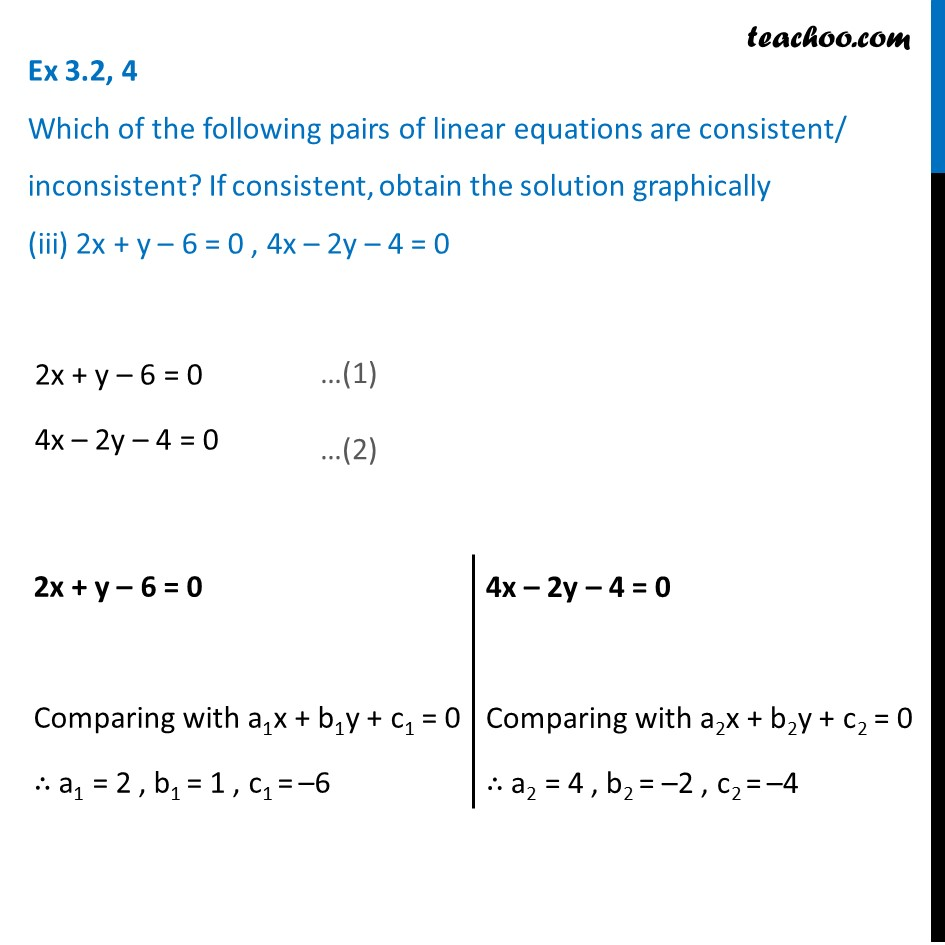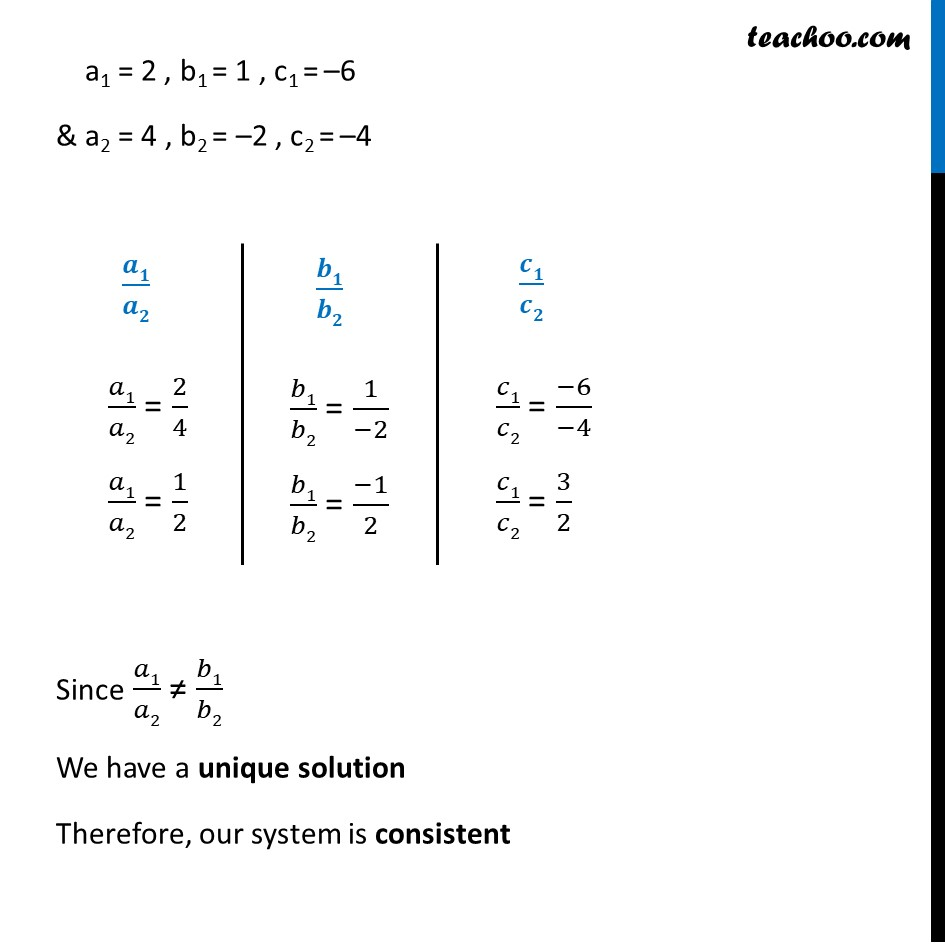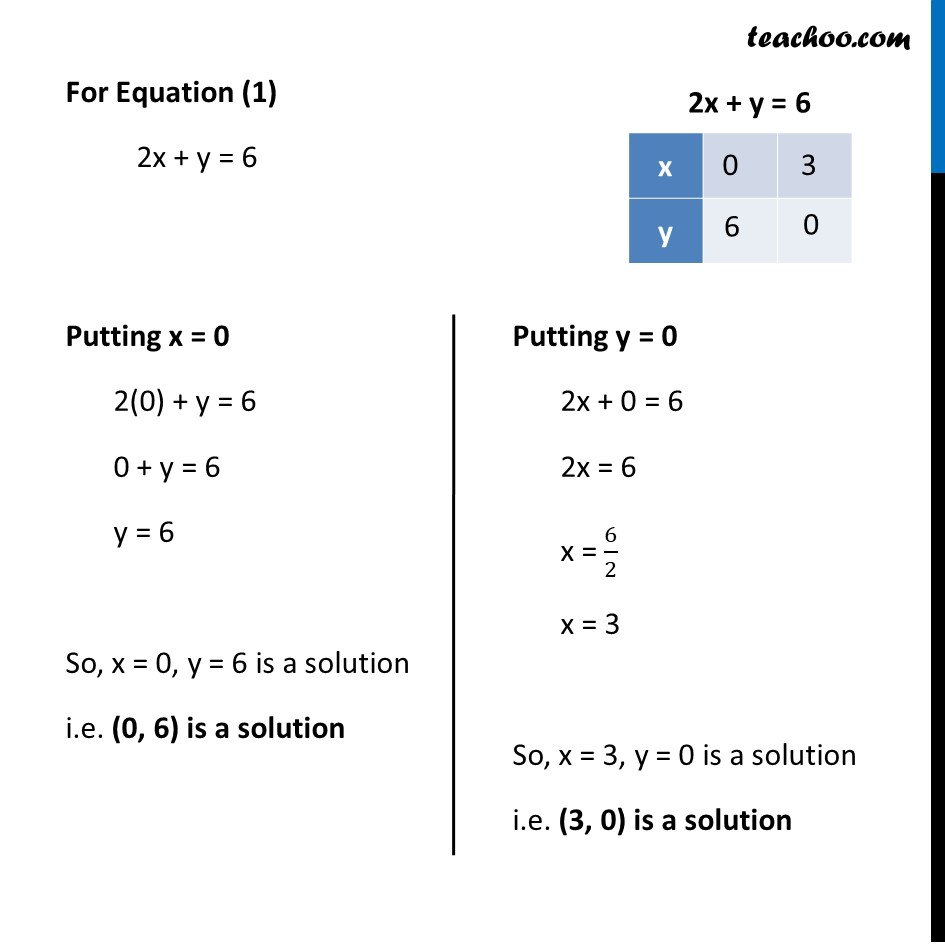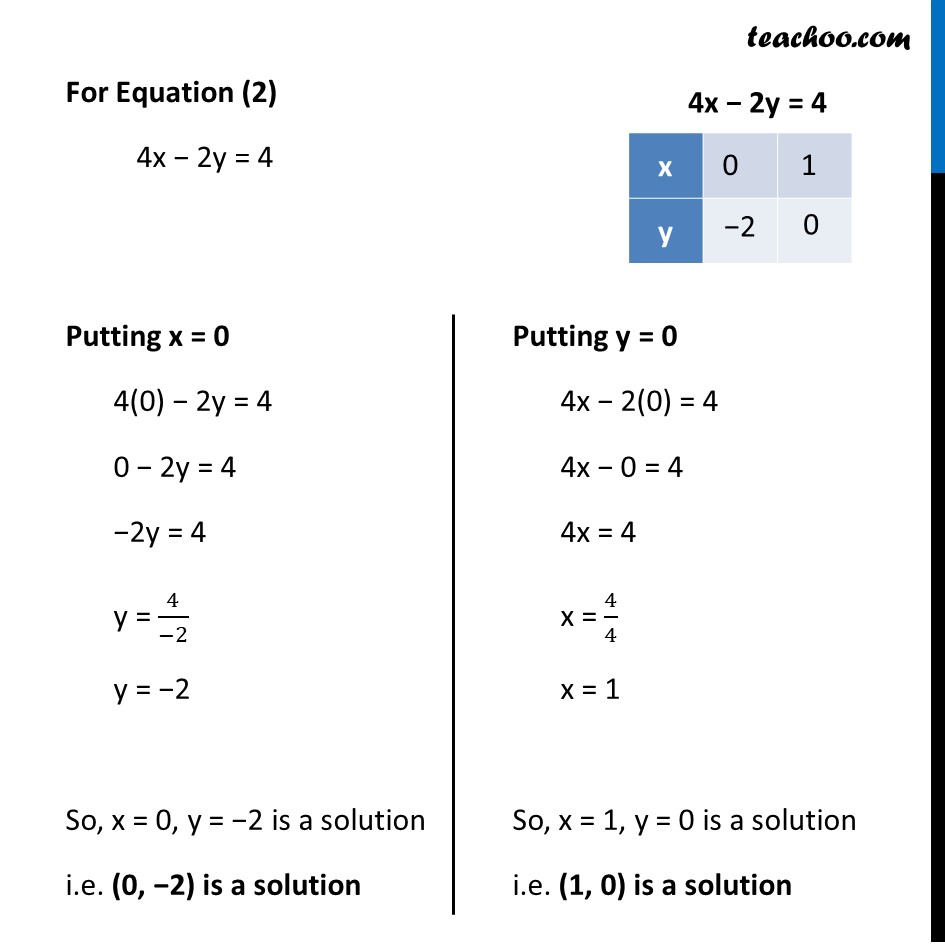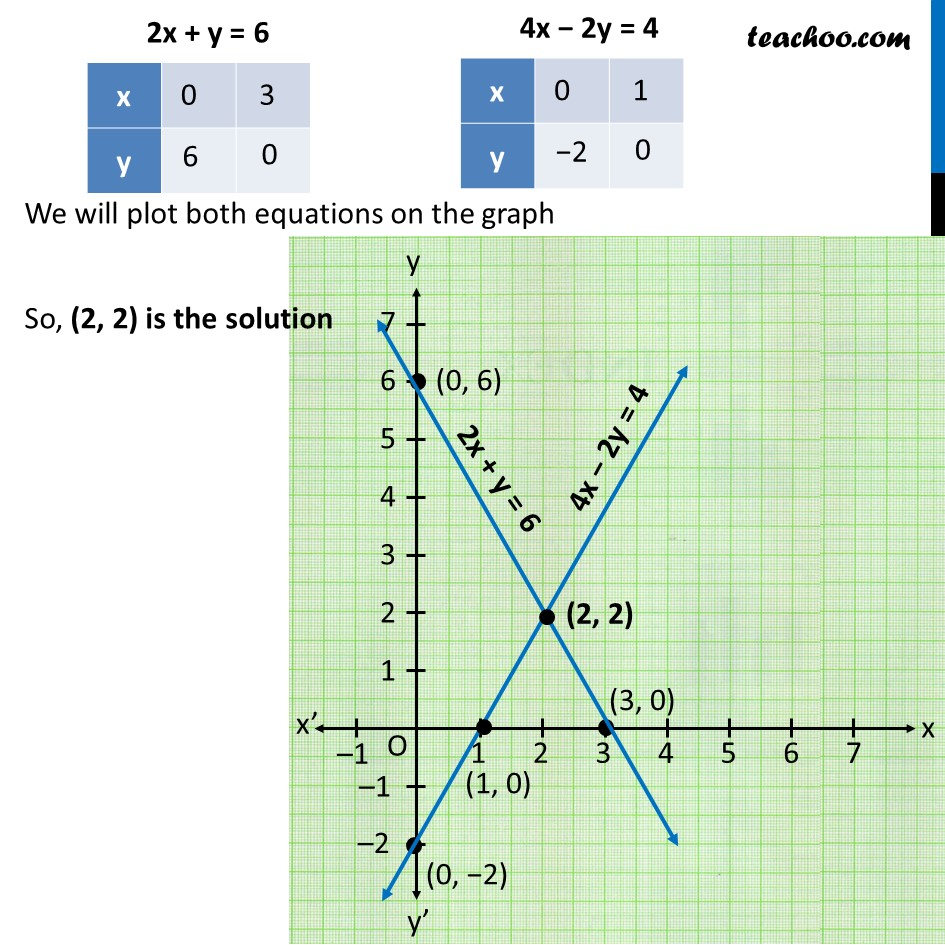Learn in your speed, with individual attention - Teachoo Maths 1-on-1 Class

### Transcript

Ex 3.1, 4 Which of the following pairs of linear equations are consistent/ inconsistent? If consistent, obtain the solution graphically (iii) 2x + y – 6 = 0 , 4x – 2y – 4 = 0 2x + y – 6 = 0 4x – 2y – 4 = 0 2x + y – 6 = 0 Comparing with a1x + b1y + c1 = 0 ∴ a1 = 2 , b1 = 1 , c1 = –6 4x – 2y – 4 = 0 Comparing with a2x + b2y + c2 = 0 ∴ a2 = 4 , b2 = –2 , c2 = –4 a1 = 2 , b1 = 1 , c1 = –6 & a2 = 4 , b2 = –2 , c2 = –4 𝒂𝟏/𝒂𝟐 𝑎1/𝑎2 = 2/4 𝑎1/𝑎2 = 1/2 𝒃𝟏/𝒃𝟐 𝑏1/𝑏2 = 1/(−2) 𝑏1/𝑏2 = (−1)/2 𝒄𝟏/𝒄𝟐 𝑐1/𝑐2 = (−6)/(−4) 𝑐1/𝑐2 = 3/2 Since 𝑎1/𝑎2 ≠ 𝑏1/𝑏2 We have a unique solution Therefore, our system is consistent For Equation (1) 2x + y = 6 Putting x = 0 2(0) + y = 6 0 + y = 6 y = 6 So, x = 0, y = 6 is a solution i.e. (0, 6) is a solution Putting y = 0 2x + 0 = 6 2x = 6 x = 6/2 x = 3 So, x = 3, y = 0 is a solution i.e. (3, 0) is a solution For Equation (2) 4x − 2y = 4 Putting x = 0 4(0) − 2y = 4 0 − 2y = 4 −2y = 4 y = 4/(−2) y = −2 So, x = 0, y = −2 is a solution i.e. (0, −2) is a solution Putting y = 0 4x − 2(0) = 4 4x − 0 = 4 4x = 4 x = 4/4 x = 1 So, x = 1, y = 0 is a solution i.e. (1, 0) is a solution We will plot both equations on the graph So, (2, 2) is the solution